# Test: Power Systems- 3

## 25 Questions MCQ Test GATE Electrical Engineering (EE) 2023 Mock Test Series | Test: Power Systems- 3

Description
Attempt Test: Power Systems- 3 | 25 questions in 75 minutes | Mock test for GATE preparation | Free important questions MCQ to study GATE Electrical Engineering (EE) 2023 Mock Test Series for GATE Exam | Download free PDF with solutions
QUESTION: 1

### A Δ -ΔT/F having rating 132KV/66KV, 50 MVA, the base impedance on primary side

Solution: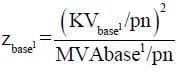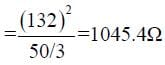QUESTION: 2

### The impedance of a Δ- Y 11000/400V 3-ΔT/F of capacity 100 KVA as on its name plate as z = (0.02 + j0.07) pu. The ohmic impedance in Ωreferred to primary (11000 V) side

Solution: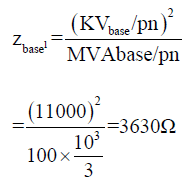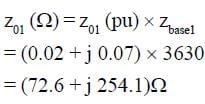QUESTION: 3

###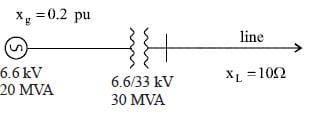take 6.6 KV, 20 MVA on G side, the pu impedance of line is

Solution: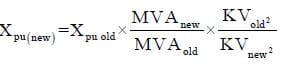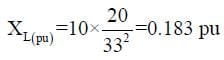QUESTION: 4

The sequence voltage of a 3φ system is given as

0.87 pu = Va1
-0.33 pu = Va0
-0.54 pu = Va2
What is the phase voltages on 11KV

Solution:

Va = Va0 + Va1 + Va2 = 0
Vb = Va0 + α2Va1 + αVa2

= -0.33 + 1 ∠240 (0.87) + (1∠120) (-0.54)
= 1.232 ∠-97.69 KV
Vc = Va0 + αVal + α2Va2
= 1.317 ∠112.60 pu

Va(KV)= 0KV
Vb (KV) = Vb (pu) x KVbasd/pm
= 8.36 KV
Vc (KV) = 8.36 KV

QUESTION: 5

An unsymmetrical fault on a transmission line gives the sequence voltage and sequence current

Va1 = 0.837 pu
Va2 = -0.45 pu
Va0 = -0.2 pu
and sequence current I1 = I2 = I0 = -j1.2 pu. What is the type of the fault.

Solution:

As all the sequence current is
same, fault is LG.

*Answer can only contain numeric values
QUESTION: 6

What is fault impedance value on the base of 11KV, 20 MVA

Solution: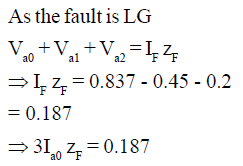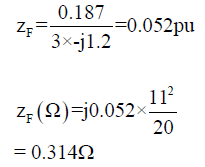QUESTION: 7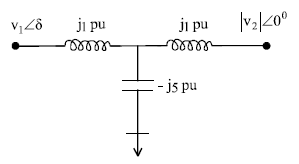|V1| = |V2| =1pu , what is the maximum power flow in this Network.

Solution: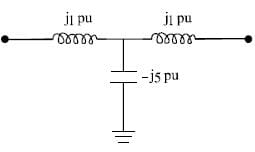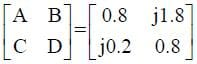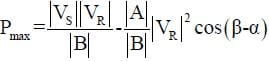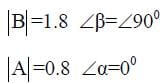= 55 pu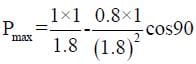Notice that without capactior pr.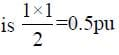QUESTION: 8

In which of the following condition the system is stable (ω is speed)

Solution:

For stability, speed must never
be continuously increase or
decrease so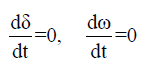QUESTION: 9

An alternator having E = 1.2 pu connected to ∞ bus through a power network. The transfer reactance between alternator and ∞ bus before fault, during fault, and after clearing fault are X1 = 0.5, X= 2.0, X3 = 1.5 pu. If initially Pm0 = Peo = 1 pu then what is δcr made by rotor such that system is stable?

Solution: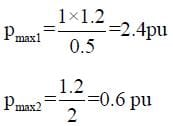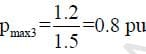as pm is 1 pu, which is greater than
pmax3, even if fault is cleared,
mech. input will always be
higher. System will remain
unstable.

QUESTION: 10

A 50 bus system with 200 transmission line. How many zeroes are in Y bus matrix.

Solution: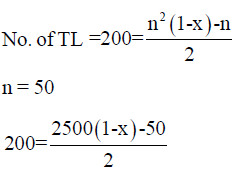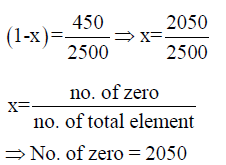QUESTION: 11

For a 3 bus network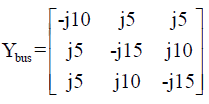a shunt capacitor of -j0.5 is added at 2nd bus, Y22 new = ?

Solution: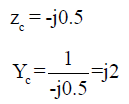z22 new = -j15 + j2 = -j13

QUESTION: 12

A Network having postive, negative and zero sequence bus impedance matrix as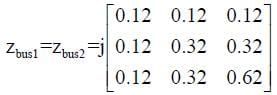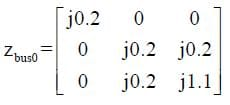what is the LG fault current at bus 2 with zf = j0.1 pu. Pre-fault voltage at bus 2 is 1.05 pu

Solution: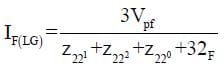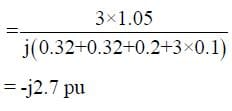QUESTION: 13

A 3φ load of 50 MW, 0.6 pf, is operating at 33KV, what is capacitance per phase required by the capacitor bank connected in Δ to improve pf to 0.8 lag.

Solution: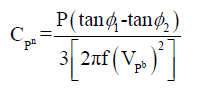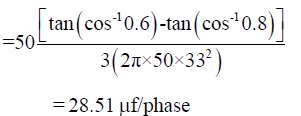QUESTION: 14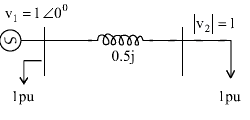what is reactive power support required at bus 2.

Solution:

As at load, no imaginary term is
given. This is active power only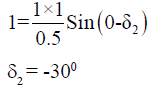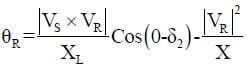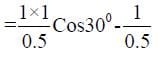= -0.267
as only active load is present a
capacitor must be installed to
get reactive power zero
so, θrequired = 0.267

QUESTION: 15

A Network containing 20 voltage controlled bus, 10 reactive power support bus, a generator bus, 5 fixed shunt capacitance bus, total voltage controlled bus is

Solution:

Shunt capacity is not voltage
controlled bus,
Total no. of voltage controlled
bus

= 20 + 10 + (9 - 1)
= 38

QUESTION: 16

A transmission line of length 300 km operating at 50Hz, the receiving end voltage under no load VS = 1pu is

Solution: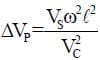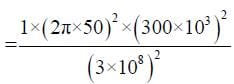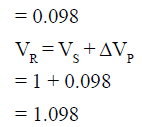QUESTION: 17

A transmission line of length 600km, has a phase shift in degree of

Solution: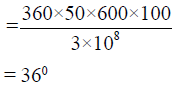QUESTION: 18

A generator having H = 6 MJ/MVA is connected to generator having H = 4 MJ/MVA. A fault reduce the pr. out put to 0.6 pu which was initially 1 pu. Find angular acceleration in elect degree/S2

Solution: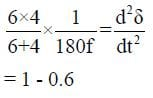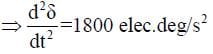QUESTION: 19

A transmission line has impedance (3 + j4) Ω/phase. The power factor of the load for maximum V.R.

Solution:

For maximum V.R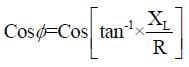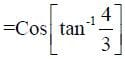= 0.6

QUESTION: 20

A 3φ transmission line has ABCD parameters as A= 0.75 ∠0° = D, B = 100∠90°Ω, C = 0.5 x 10-6 ∠90° ℧ operating at Vs = 200 KV, VR at no load, and shunt reactor for compensating Ferranti-effect

Solution: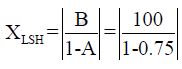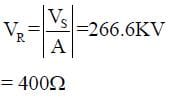*Answer can only contain numeric values
QUESTION: 21

In previous question, the charging current at no load is_____

Solution: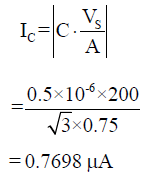QUESTION: 22

A TL has zS = 400Ω is terminated with a cable with zL = 50Ω. A rectangular pulse of 100KV is travelling towards the cable. The transmitted volt will be

Solution: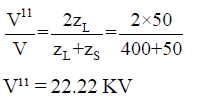QUESTION: 23

A 3φ transmission line having a surge impedance of 400Ω is operating at 400 KV line is compensated with 30% of shunt capacitor at the load end. The other end is connected to a generator which is delivering power at 200 MW. SIL of the above system is

Solution: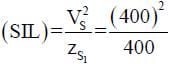= 400 MW

QUESTION: 24

In above question, SIL after compensation is

Solution: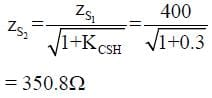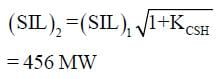QUESTION: 25

A TL supported by 3 insulation disc, the maximum voltage of each disc is 25 KV. Find the operating voltage per phase of the line given efficiency η = 78%

Solution: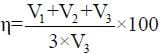as (V1 + V2 + V3) is per phase voltage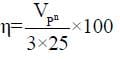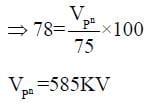Use Code STAYHOME200 and get INR 200 additional OFF Use Coupon Code